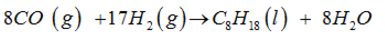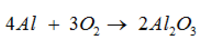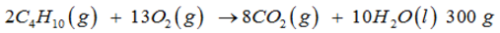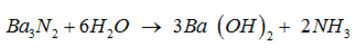Courses

# Basic Concepts Of Chemistry - Practice Test (1)

## 25 Questions MCQ Test Additional Question Bank | Basic Concepts Of Chemistry - Practice Test (1)

Description
This mock test of Basic Concepts Of Chemistry - Practice Test (1) for Class 9 helps you for every Class 9 entrance exam. This contains 25 Multiple Choice Questions for Class 9 Basic Concepts Of Chemistry - Practice Test (1) (mcq) to study with solutions a complete question bank. The solved questions answers in this Basic Concepts Of Chemistry - Practice Test (1) quiz give you a good mix of easy questions and tough questions. Class 9 students definitely take this Basic Concepts Of Chemistry - Practice Test (1) exercise for a better result in the exam. You can find other Basic Concepts Of Chemistry - Practice Test (1) extra questions, long questions & short questions for Class 9 on EduRev as well by searching above.
QUESTION: 1

### Chemistry does not play a major role in

Solution:

Chemistry does not deal in explaining superconductivity.

QUESTION: 2

### One mole is the amount of a substance that contains as many particles or entities as there are atoms in

Solution:

One mole is the amount of a substance that contains as many particles or entities as there are atoms in exactly 12 g of the 12C isotope

QUESTION: 3

### ....KOH +.... H3PO4 →.... K3PO4 + ....H2O.. This equation can be balanced by inserting the following in blank spaces

Solution:

3KOH + H3PO4 → K3PO4 + 3H2O.

QUESTION: 4

We have to prepare a solution of 0.2 M NaOH from the available 1 M solution that is given to us. How much volume of 1M NaOH is required to be taken which contains 0.2 moles of NaOH

Solution:

M1V1 =M2V2 where M1M1 = 1M V1=?

QUESTION: 5

What is the mass of 1 molecule of O2?

Solution:
QUESTION: 6

Matter can be classified at the macroscopic or bulk level as

Solution:

at macroscopic level matter is classified as mixture or pure substances.

QUESTION: 7

A given compound always contains exactly the same proportion of elements by weight. This law is stated by

Solution:

this law is stated by Proust.

QUESTION: 8

-----CO (g) + ----- H2(g) → ----- C8H18(l) + ----- H2O. This equation can be balanced by inserting the following in blank spaces

Solution:QUESTION: 9

There are ____ in 0.101 mm?

Solution:

1cm=10mm

QUESTION: 10

What is the mass of 1 mole of O2?

Solution:

mass of 6.02 x 1023 molecules of O2 = 32g = mass of 1mole of O2.

QUESTION: 11

In a homogeneous mixture,

Solution:

Homogeneous mixture has a uniform composition throughout.

QUESTION: 12

Solution:

Avagadros constant is entities in 1 mol = 6.022 x 1023

QUESTION: 13

---- Al + ------ O2 → ------ Al2O3 . This equation can be balanced by inserting the following in blank spaces

Solution:QUESTION: 14

20 m/s is the same as:

Solution:

1km = 1000m

QUESTION: 15

For the reactionof C4H10 is combusted in 1000 g of O2. The yield of H2OH2O and the limiting agent in the reaction are

Solution:

moloes of C4H, given = 51.72 mol Moles of O2 = 31.25 mol. Since 2 mol ofC4H10 requires 13 mol of O2 so 51.72 mol of C4H10 require 336.18 mol of O2 but O2 we have is only 31.25 mol therefore O2 is limiting reagent. Therefore 13 mol of O2 gives 10 mol of H2O so 31.25 mol will give 24.038 mol of water = 432 g of water.

QUESTION: 16

Which of the following statements about pure substances correct?

Solution:

pure substances are elements and compounds . they have fixed composition while mixtures have variable composition.

QUESTION: 17

An empirical formula represents the simplest

Solution:

empirical formula is the simplest formula of a compound.

QUESTION: 18

------ Ba3N2 +----- H2O → ------ Ba (OH)2 + ------ NH3 . This equation can be balanced by inserting the following in blank spaces

Solution:QUESTION: 19

30 microliters is the same as:

Solution:
QUESTION: 20

How many molecules of H2O are there in 18g of water? (Hint: Avagadro’s Number = 6.02 x 1023 atoms/mol)

Solution:

18g H2O = 1mol water = 6.02 x 1023 molecules of water

QUESTION: 21

Particles of compounds are

Solution:

compounds are made when molecules or atoms of two or more different elements combine together in fixed ratio.

QUESTION: 22

Molecular formula shows the.

Solution:

Molecular formula is the exact no. of atoms present in a molecule of a compound.

QUESTION: 23

The molar mass of CO2 is

Solution:

Molar mass= 12+2(16)=44g

QUESTION: 24

20 grams is the same as:

Solution:

1mg=1000

QUESTION: 25

78 g of an optically active L-amino acid (A) is treated with NaNO2/HCl at 0º C. 448 cm3 of nitrogen was at STP is evolved. A sample of protein has 0.25% of this amino acid by mass. The molar mass of the protein is

Solution: